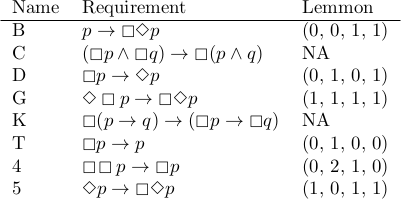# Names and numbers for modal logic axioms

Stanislaw Ulam once said

Using a term like nonlinear science is like referring to the bulk of zoology as the study of non-elephant animals.

There is only one way to be linear, but there are many ways to not be linear.

A similar observation applies to non-classical logic. There are many ways to not be classical.

## Modal logic axioms

Modal logic extends classical logic by adding modal operators □ and ◇. The interpretation of these operators depends on the application, but a common interpretation is “necessarily” and “possibly.”

There are a bewildering number of possible axioms to choose from in order to create a system of modal logic, and these often have cryptic names like “K”, “G”, and “5”. Seldom is any reason given for the names, and after digging into this a little, I think I know why: There isn’t much reason. Or rather, the reasons are historical rather than mnemonic.

For example, Axiom T is so named because Kurt Gödel published a paper that called some set of axioms “System T.” Of course that raises the question of why Gödel called his system “T.”

Axiom 5 gets its name from C. I. Lewis’ classification of logic systems. But Lewis’ numbering of systems S1 through S5 was essentially arbitrary, other than larger numbers corresponding to more axioms.

Unless you’re interested in the history for its own sake, it’s probably not worth questioning why rules are called what they are.

## Lemmon numbering

Although there’s not much logic behind the names of model logic axioms, there is a convenient way to catalog many of them. E. J. Lemmon  observed that many axioms for modal logic can be written in the formHere’s a list of some common modal logic axioms, along with their names and Lemmon numbers.E. J. Lemmon, An Introduction to Modal Logic, American Philosophical Quarterly, Monograph 11. Oxford, 1977.